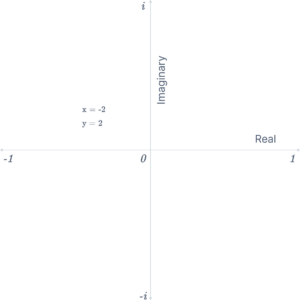# Complex Number Calculator

The Complex Number Calculator is a versatile online tool designed to facilitate seamless operations between sets of complex numbers. This powerful calculator evaluates complex number expressions and performs fundamental arithmetic, empowering users with quick and accurate results.

First number: $$z_1 = a + bi$$
First number: $$z_2 = c + di$$

## What are Complex numbers?

An expression of the form $$a + bi$$, where a and b are real values, is a complex number. For example, if z is a complex number and $$z = a + bi$$, then a and b are the real and imaginary parts of z.

Numerous scientific disciplines, including engineering, electromagnetism, quantum physics, applied mathematics, and chaos theory, require complex numbers.

Either part of a complex number can be equal to zero. When the imaginary part (bi) is zero, the number reduces to a pure real number (a), and when the real part (a) is zero, it becomes a pure imaginary number (bi). This flexibility allows complex numbers to encompass all real and imaginary numbers within their domain.

 Complex Number Real Part Imaginary Part Comments 5 + 4i 5 4 3 3 0 Purely Real -4i 0 -4 Purely Imaginary

## Graphical Representation of Complex Numbers

In the following graph, observe the depiction of complex numbers along the axes. The x-axis represents the real part, while the y-axis corresponds to the imaginary part.## How to Use the Complex Number Calculator?

The complex number calculator should be used as follows:

1. Fill in the appropriate input field with the complex numbers.
2. To obtain the outcome, press the “Calculate” button now.
3. Lastly, you will get two complex numbers’ multiplication, division, addition, or subtraction.

Whether you’re a mathematics enthusiast, a student, or a professional in the scientific field, the Complex Number Calculator is your go-to tool for accurate and efficient complex number operations.

## Basic operations with complex numbers

Working with complex numbers is simple because you may utilize the imaginary unit i as a variable. And to make complicated expressions simpler, use the formula $$i^2 = -1$$. Numerous procedures are identical to those performed on two-dimensional vectors.

Combine the imaginary portions (with i) and the real parts (without i):

Use rule $$(a + bi) + (c + di) = (a + c) + (b + d)i$$ is equivalent to this.

$$(1 + i) + (7 – 5i) = 8 – 4i$$

Example: Addition of Complex Numbers (6 + 3i) and (2 + 5i)

Step 1: We add the real parts (6 and 2) to get the real part of the result, which is 6 + 2 = 8.

Step 2: Next, we add the imaginary parts (3i and 5i) to get the imaginary part of the result, which is 3 + 5 = 8.

Finally, we combine the real and imaginary parts and write the complex number in standard form to obtain the final result: 8 + 8i, representing the sum of the two complex numbers (6 + 3i) and (2 + 5i).

### Subtraction

Subtract the imaginary components (with i) and the real parts again, very simply:

Use rule $$(a + bi) + (c + di) = (a – c) + (b – d)i$$ is equivalent to this.

$$(10 – 5i) – (-6 + 5i) = 16 – 10i$$

### Multiplication

You can use this formula for multiplication between two complex numbers, $$z_1$$ and $$z_2$$

$$z_1 = a + bj$$

$$z_2 = c + dj$$

Multiplication Formula

$$= z_1 × z_2$$

$$= (a + bj) × (c + dj)$$

$$= ac + adj + bcj + bdj^2$$

But $$j^2 = -1$$,

So $$z_1 × z_2 = (ac – bd) + (bc + ad)j$$

Example: Multiplication of Complex Numbers (4 + 5i) and (2 + 3i)

Step 1: Multiply the real parts (4 and 2):
Real Part: $$4 × 2 = 8 ) Step 2: Multiply the imaginary parts (5i and 3i): Imaginary Part: \( 5i × 3i = 15i^2$$

Step 3: Simplify the imaginary part using the fact that $$i^2 = -1$$:
Imaginary Part: $$15i^2 = -15$$

Step 4: Multiply the real part and imaginary part of the two complex numbers:
Real and Imaginary Part: (4 + 5i)(2 + 3i) = (4 × 2) + (4 × 3i) + (5i × 2) + (5i × 3i) = 8 + 12i + 10i + (-15)

Step 5: Combine like terms to write the complex number in standard form:
Real and Imaginary Part: 8 + 12i + 10i – 15 = -7 + 22i

Finally, multiplying the complex numbers (4 + 5i) and (2 + 3i) is -7 + 22i.

## FAQs

How to write complex numbers in standard form?

The complex numbers will be expressed as $$a + bi$$ in standard form, where a represents the real part and bi represents the imaginary part. A complex number is something like $$3 + 5i$$. The real portion is 3, and the imaginary portion is $$5i$$.

What is the modulus of complex numbers?

The distance of a complex number from its origin in the argand plane is known as the modulus of the complex number. If $$z = x + iy$$ is a complex number with x and y are real and $$i = \sqrt{-1}$$, then the non-negative value $$\sqrt{x^2 + y^2}$$ is the modulus of the complex number $$z = x + iy$$.